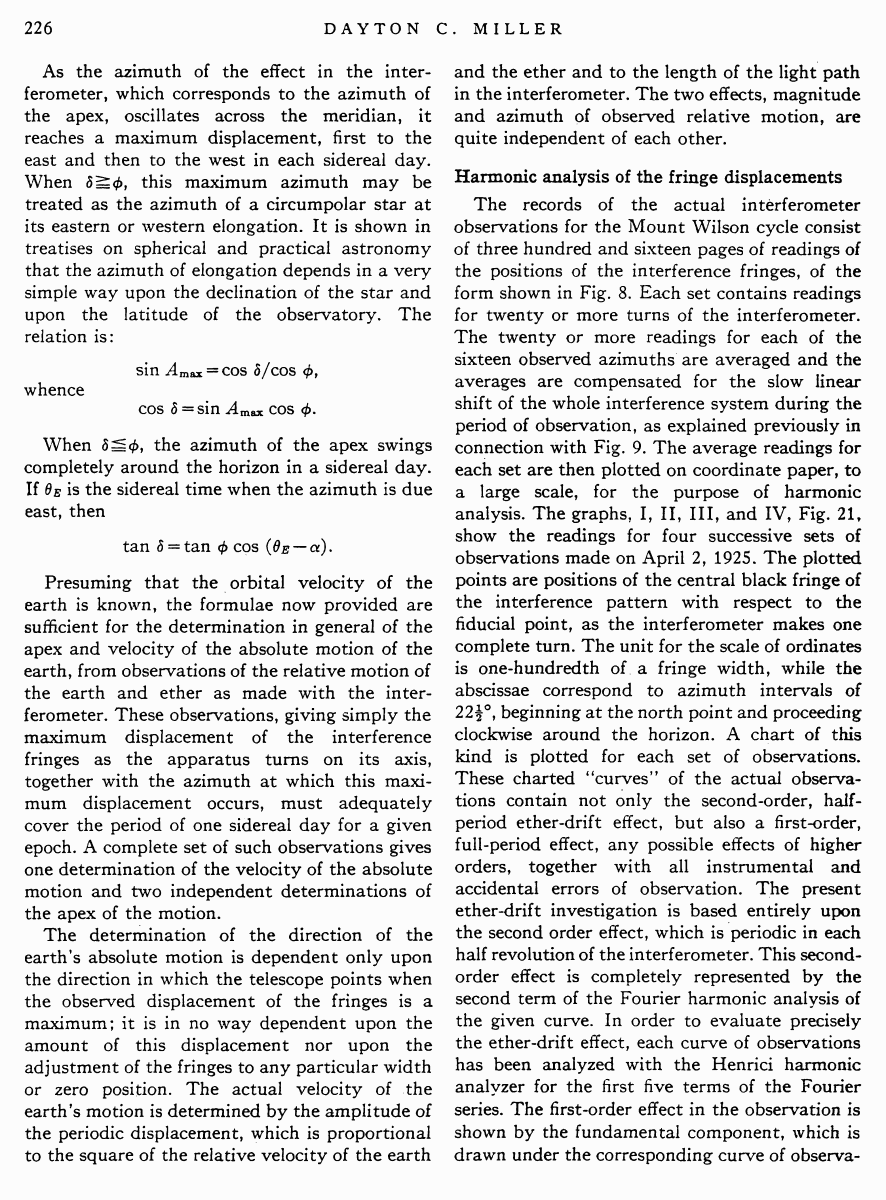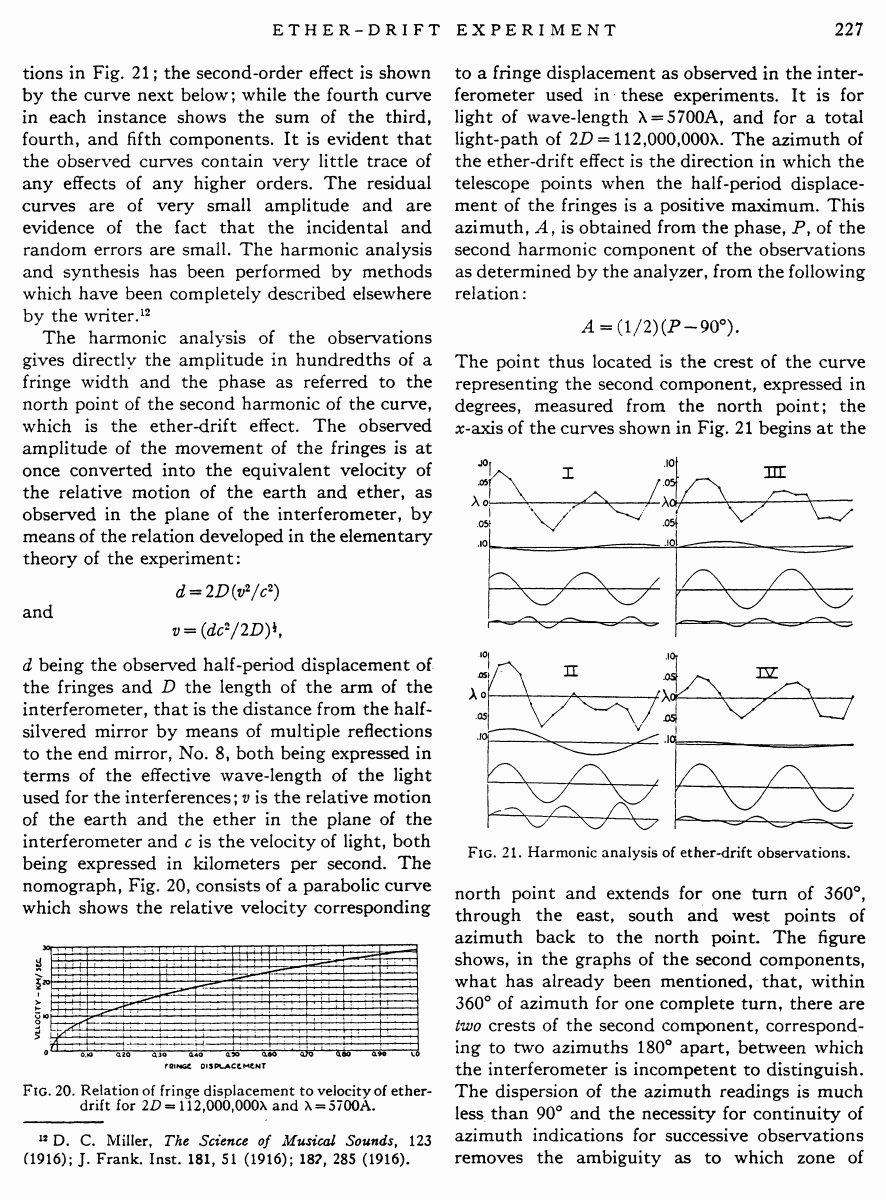# Miller D.C. The Ether-Drift Experiment and the Determination of the Absolute Motion of the Earth // Reviews of modern physics, Vol.5, July 1933

Start   PDF   <<<  Page 226   >>>203  204  205  206  207  208  209  210  211  212  213  214  215  216  217  218  219  220  221  222  223  224  225  226 227  228  229  230  231  232  233  234  235  236  237  238  239  240  241  242 As the azimuth of the effect in the interferometer, which corresponds to the azimuth of the apex, oscillates across the meridian, it reaches a maximum displacement, first to the east and then to the west in each sidereal day. When δ ≥ φ, this maximum azimuth may be treated as the azimuth of a circumpolar star at its eastern or western elongation. It is shown in treatises on spherical and practical astronomy that the azimuth of elongation depends in a very simple way upon the declination of the star and upon the latitude of the observatory. The relation is: sin Amax = cos δ /cos φ, whence cos δ = sin Amax cos φ. When δ ≤ φ, the azimuth of the apex swings completely around the horizon in a sidereal day. If θE is the sidereal time when the azimuth is due east, then tan δ = tan φ cos (θE ¢α). Presuming that the orbital velocity of the earth is known, the formulae now provided are sufficient for the determination in general of the apex and velocity of the absolute motion of the earth, from observations of the relative motion of the earth and ether as made with the interferometer. These observations, giving simply the maximum displacement of the interference fringes as the apparatus turns on its axis, together with the azimuth at which this maximum displacement occurs, must adequately cover the period of one sidereal day for a given epoch. A complete set of such observations gives one determination of the velocity of the absolute motion and two independent determinations of the apex of the motion. The determination of the direction of the earthÆs absolute motion is dependent only upon the direction in which the telescope points when the observed displacement of the fringes is a maximum; it is in no way dependent upon the amount of this displacement nor upon the adjustment of the fringes to any particular width or zero position. The actual velocity of the earthÆs motion is determined by the amplitude of the periodic displacement, which is proportional to the square of the relative velocity of the earth and the ether and to the length of the light path in the interferometer. The two effects, magnitude and azimuth of observed relative motion, are quite independent of each other. Harmonic analysis of the fringe displacements The records of the actual interferometer observations for the Mount Wilson cycle consist of three hundred and sixteen pages of readings of the positions of the interference fringes, of the form shown in Fig. 8. Each set contains readings for twenty or more turns of the interferometer. The twenty or more readings for each of the sixteen observed azimuths are averaged and the averages are compensated for the slow linear shift of the whole interference system during the period of observation, as explained previously in connection with Fig. 9. The average readings for each set are then plotted on coordinate paper, to a large scale, for the purpose of harmonic analysis. The graphs, I, II, III, and IV, Fig. 21, show the readings for four successive sets of observations made on April 2, 1925. The plotted points are positions of the central black fringe of the interference pattern with respect to the fiducial point, as the interferometer makes one complete turn. The unit for the scale of ordinates is one-hundredth of a fringe width, while the abscissae correspond to azimuth intervals of 221/2░, beginning at the north point and proceeding clockwise around the horizon. A chart of this kind is plotted for each set of observations. These charted ōcurvesö of the actual observations contain not only the second-order, halfperiod ether-drift effect, but also a first-order, full-period effect, any possible effects of higher orders, together with all instrumental and accidental errors of observation. The present ether-drift investigation is based entirely upon the second order effect, which is periodic in each half revolution of the interferometer. This second-order effect is completely represented by the second term of the Fourier harmonic analysis of the given curve. In order to evaluate precisely the ether-drift effect, each curve of observations has been analyzed with the Henrici harmonic analyzer for the first five terms of the Fourier series. The first-order effect in the observation is shown by the fundamental component, which is drawn under the corresponding curve of observa tions in Fig. 21; the second-order effect is shown by the curve next below; while the fourth curve in each instance shows the sum of the third, fourth, and fifth components. It is evident that the observed curves contain very little trace of any effects of any higher orders. The residual curves are of very small amplitude and are evidence of the fact that the incidental and random errors are small. The harmonic analysis and synthesis has been performed by methods which have been completely described elsewhere by the writer.12 The harmonic analysis of the observations gives directly the amplitude in hundredths of a fringe width and the phase as referred to the north point of the second harmonic of the curve, which is the ether-drift effect. The observed amplitude of the movement of the fringes is at once converted into the equivalent velocity of the relative motion of the earth and ether, as observed in the plane of the interferometer, by means of the relation developed in the elementary theory of the experiment: d = 2D(v2/c2) and v = (dc2/2D)1/2, d being the observed half-period displacement of the fringes and D the length of the arm of the interferometer, that is the distance from the halfsilvered mirror by means of multiple reflections to the end mirror, No. 8, both being expressed in terms of the effective wave-length of the light used for the interferences; v is the relative motion of the earth and the ether in the plane of the interferometer and c is the velocity of light, both being expressed in kilometers per second. The nomograph, Fig. 20, consists of a parabolic curve which shows the relative velocity corresponding Fig. 20. Relation of fringe displacement to velocity of ether-drift for 2D = 112,000,000λ and λ = 5700A. 12 D. C. Miller, The Science of Musical Sounds, 123 (1916); J. Frank. Inst. 181, 51 (1916); 182, 285 (1916). to a fringe displacement as observed in the interferometer used in these experiments. It is for light of wave-length λ = 5700A, and for a total light-path of 2D = 112,000,000λ. The azimuth of the ether-drift effect is the direction in which the telescope points when the half-period displacement of the fringes is a positive maximum. This azimuth, A, is obtained from the phase, P, of the second harmonic component of the observations as determined by the analyzer, from the following relation: A = (1/2) (P ¢ 90░). The point thus located is the crest of the curve representing the second component, expressed in degrees, measured from the north point; the x-axis of the curves shown in Fig. 21 begins at the Fig. 21. Harmonic analysis of ether-drift observations. north point and extends for one turn of 360░, through the east, south and west points of azimuth back to the north point. The figure shows, in the graphs of the second components, what has already been mentioned, that, within 360░ of azimuth for one complete turn, there are two crests of the second component, corresponding to two azimuths 180░ apart, between which the interferometer is incompetent to distinguish. The dispersion of the azimuth readings is much less than 90░ and the necessity for continuity of azimuth indications for successive observations removes the ambiguity as to which zone of